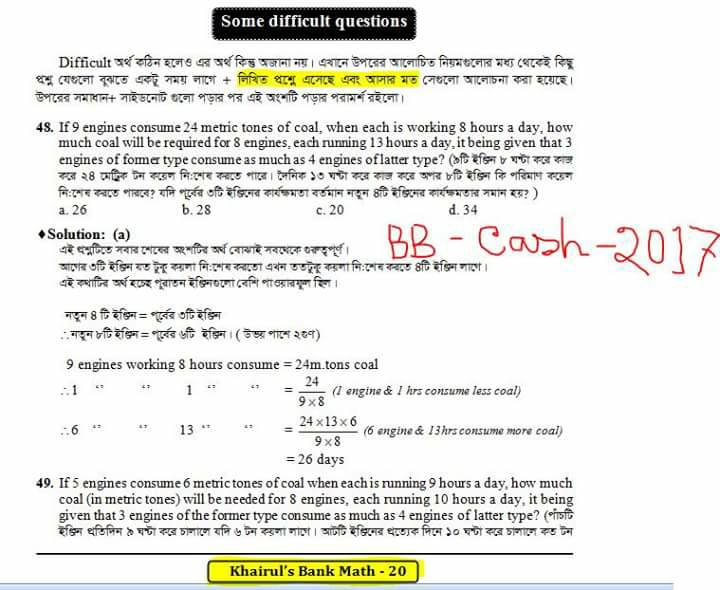# Bangladesh Bank Cash officer job Exam Solution 2017

Bangladesh Bank Cash officer job Exam was held and We published BB Cash Officer Math Question Solution 2017. Let`s Check Below Bangladesh Bank Cash officer Exam Solution in February 2017.

## Bangladesh Bank Cash officer Exam Solution 2017

Written Math :

1. Shakil started a business investing Tk. 25,000 in 2009, In 2010, he invested an additional amount of Tk. 10,000 and Raihan joined him with an amount of Tk. 35,000. In 2011, Shakil invested another additional amount of Tk. 10,000 and Jafor joined them with an amount of Tk. 35,000. What will be Raihan’s share in the profit of Tk. 1,50,000 earned at the end of 3 years from the start of the business in 2009?

.
Solution:
In 3 years,
Shakil’s investment = Tk. (25,000×3 + 10,000×2 + 10,000×1) = Tk. 105000
Raihan’s investment = Tk.(35000×2) = Tk. 70000
Jafor’s investment = Tk.(35000×1) = Tk. 35000
Their investment ratio,
Shakil : Raihan : Jafor = 105000 : 70000 : 35000 = 3:2:1
Sum of the ratios = 3+2+1 =6
So, Raihan’s share = Tk. 150000×2/6 = Tk. 50000 (Ans.)
======
2. If 9 engines consume 24 metric tonnes of coal, when each is working 8 hours day, how much coal will be required for 8 engines, each running 13 hours a day, it being given that 3 engines of former type consume as much as 4 engines of latter type?
.
Solution:
Given that
4 engines of latter type = 3 engines of former type
So 8 engines of latter type = (3/4)×8 = 6 engines
Now,
In 8 hours 9 engines consume 24 metric tones
In 13 hours 6 engines consume (24×13×6)/8×9 metric tones = 26 metric tones (Ans.)
======
3. Dawood invested certain amount in three different schemes A, B and C with the rate of interest 10% p.a., 12% p.a. and 15% p.a. respectively. If the total interest accrued in one year was Rs. 3200 and the amount invested in Scheme C was 150% of the amount invested in Scheme A and 240% of the amount invested in Scheme B, what was the amount invested in Scheme B?
.
Solution:
Let, the amount invested in Scheme B = Tk.100x
So, the amount invested in Scheme C = Tk.(100x ×240%) = Tk. 240x
Here,
The amount invested in Scheme C = 150% of the amount invested in Scheme A
Or, Tk. 240x = 150×A/100
Or, A = Tk. 24000/150 = Tk. 160x
So, the amount invested in Scheme A = Tk.160x
Now,
Interest from Scheme A = Tk. (160x×10%) = Tk.16x
Interest from Scheme B = Tk. (100x×12%) = Tk.12x
Interest from Scheme C = Tk. (240x×15%) = Tk.36x
According to the question,
16x +12x + 36x = 3200
Or, 64x = 3200
Or, x = 3200/64
Or, 100x =50×100
Or, 100x = 5000
So, the amount invested in Scheme B = Tk. 5000(Ans.)

## Cash officer job Exam Solution 2017Hope You Read Bank Cash officer Exam Solution from our website. For Read Next Bank Job Exam Question Solution Stay visit us.

##### You May Like Also :
পোস্টটি শেয়ার দিন..